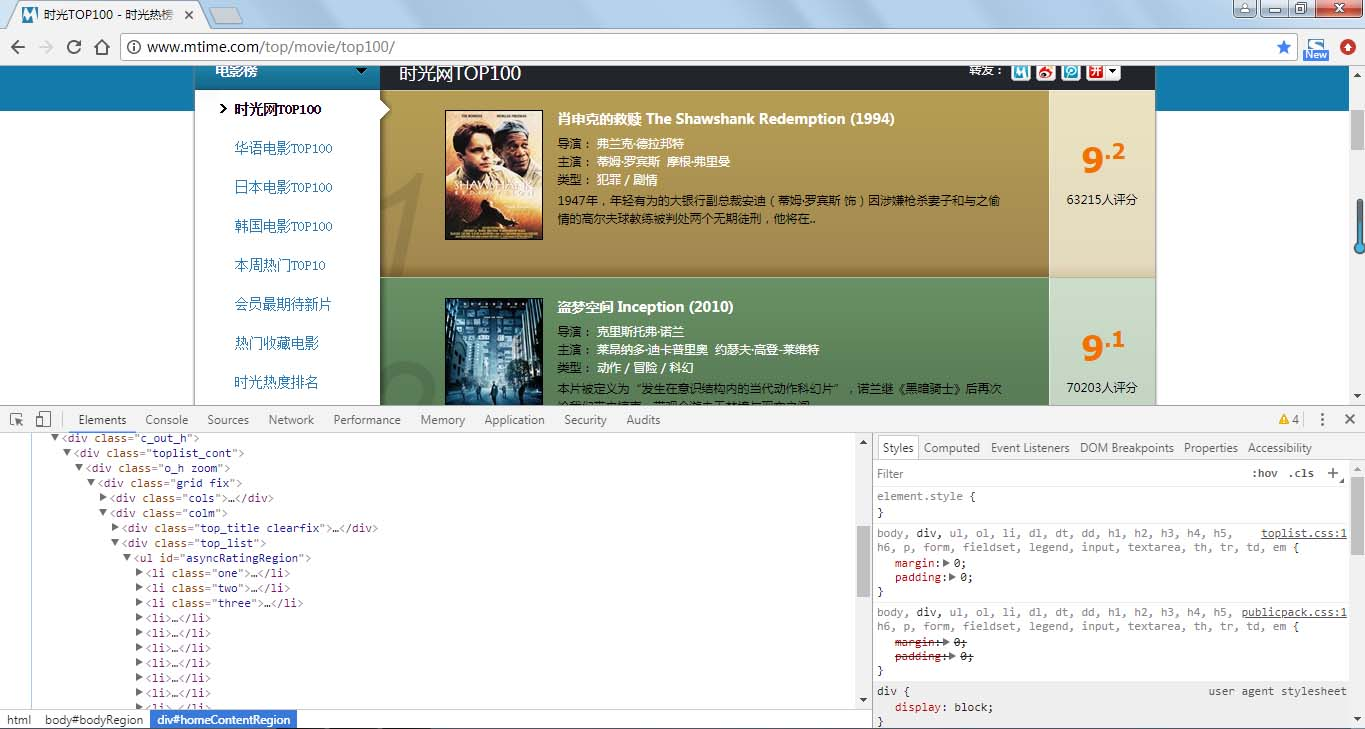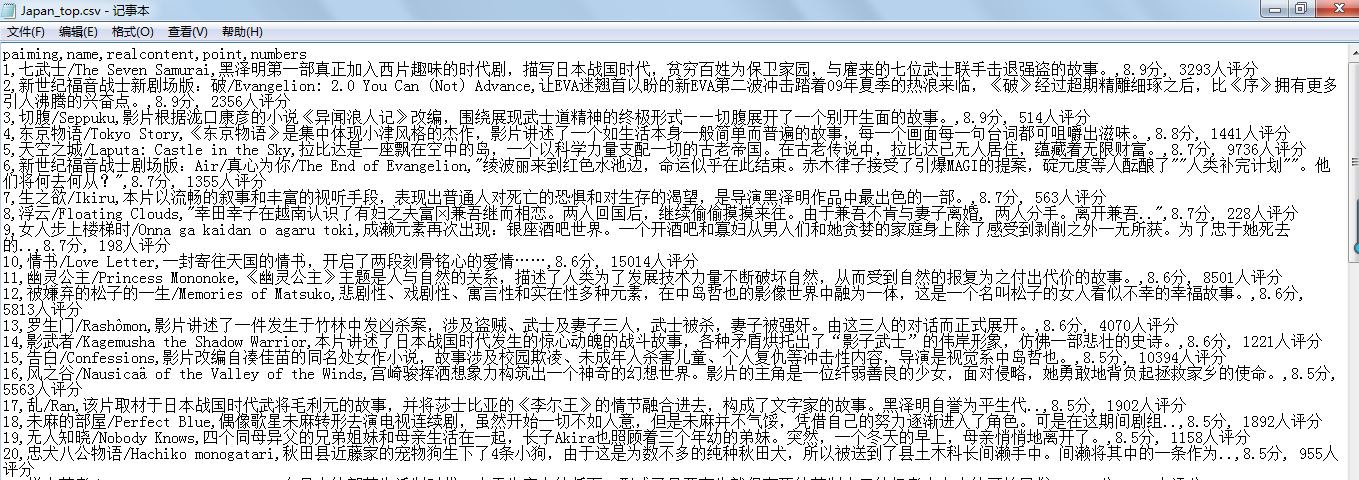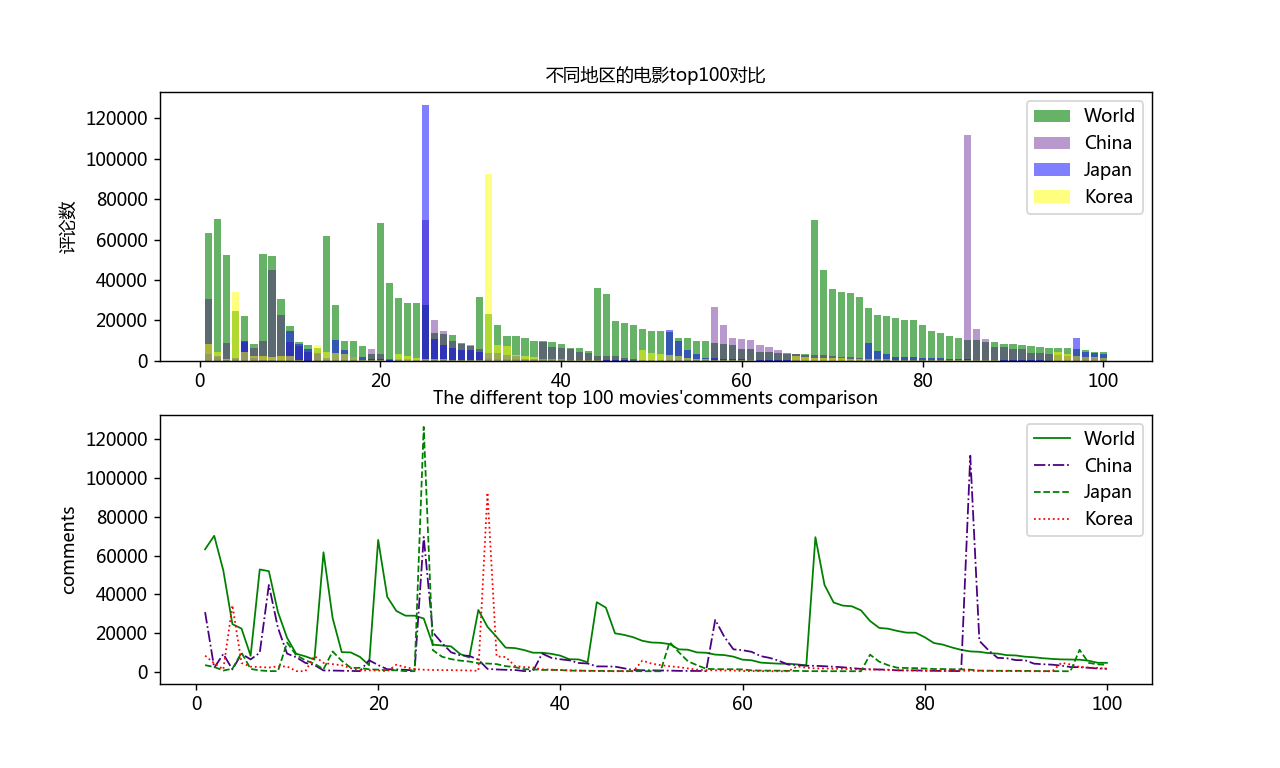python使用BeautifulSoup与正则表达式爬取时光网不同地区top100电影并对比

更新时间：2019年04月15日 09:51:53   作者：Berryguo我要评论

1.爬取时光网top100电影，华语top100电影，日本top100电影，韩国top100电影的排名情况，电影名字，电影简介，评分及评价人数

2. 将爬取数据保存为csv格式后，取出并使用matplotlib绘图库分析对比评论人数一项

3.将结果图像保存```import requests
from bs4 import BeautifulSoup
import re
import csv

#定义爬取函数
def get_infos(htmls, csvname):
#信息头
'User-Agent': 'Mozilla/5.0 (Windows NT 6.1; Win64; x64) AppleWebKit/537.36 (KHTML, like Gecko) Chrome/65.0.3325.181 Safari/537.36'
}
#flag在写入文件时判断是否为首行
flag = True
#判断第一页网址，第二页及其后的网址
for i in range(10):
if i == 0:
html = htmls
else:
html = htmls + 'index-{}.html'.format(str(i+1))
soup = BeautifulSoup(res.text, 'lxml')
alls = soup.select('#asyncRatingRegion > li') #选取网页的li节点的内容
#对节点内容进行循环遍历
for one in alls:
paiming = one.div.em.string #排名
names = str(one.select('div.mov_pic > a')) #电影名称并将列表字符串化
name = re.findall('.*?title="(.*?)">.*?', names, re.S) #使用正则表达式提取内容
content = str(one.select('div.mov_con > p.mt3')) #评论
realcontent = re.findall('.*?mt3">(.*?)</p>', content, re.S) #同上
p1 = one.find(name='span', attrs={'class': 'total'}, text=re.compile('\d')) #评分在两个节点，
p2 = one.find(name='span', attrs={'class': 'total2'}, text=re.compile('.\d'))
#判断评分是否为空
if p1 and p2 != None:
p1 = p1.string
p2 = p2.string
else:
p1 = 'no'
p2 = ' point'
point = p1 + p2 + '分'
numbers = one.find(text=re.compile('评分')) #评分数量
# 保存为csv
csvnames = 'C:\\Users\lenovo\Desktop\\' + csvname + '.csv'
with open(csvnames, 'a+', encoding='utf-8') as f:
writer = csv.writer(f)
if flag:
writer.writerow(('paiming', 'name', 'realcontent', 'point', 'numbers'))
writer.writerow((paiming, name, realcontent, point, numbers))
flag = False

#调用函数
Japan_html = 'http://www.mtime.com/top/movie/top100_japan/'
csvname1 = 'Japan_top'
get_infos(Japan_html, csvname1)

Korea_html = 'http://www.mtime.com/top/movie/top100_south_korea/'
csvname2 = 'Korea_top'
get_infos(Korea_html, csvname2)```-----------------------------------------------------------------------------------------------------------------------------------------------------------------

```import csv
from matplotlib import pyplot as plt
#中文乱码处理
plt.rcParams['font.sans-serif'] =['Microsoft YaHei']
plt.rcParams['axes.unicode_minus'] = False

csvfile_name = 'C:\\Users\lenovo\Desktop\\' + csvname + '.csv'
#打开文件并存入列表
with open(csvfile_name,encoding='utf-8') as f:
name = []
name.append(row)
#取列表中非空元素
real = []
for i in name:
if len(i) != 0:
real.append(i)
#去除中文并将数据转换为整形
t = 0
ss = []
for j in real:
ss.append(int(real[t][:-5]))
t += 1
return ss

#绘制对比图形
shu = list(range(1,101))
fig = plt.figure(dpi=128, figsize=(10, 6)) #设置图形界面
plt.subplot(2,1,1)
plt.bar(shu ,All_plt, align='center', color='green', label='World', alpha=0.6) #绘制条图形,align指定横坐标在中心，颜色，alpha指定透明度
plt.bar(shu ,China_plt, color='indigo', label='China', alpha=0.4) #绘制图形，颜色， label属性用于后面使用legend方法时显示图例标签
plt.bar(shu ,Japan_plt, color='blue', label='Japan',alpha=0.5) #绘制图形，颜色，
plt.bar(shu ,Korea_plt, color='yellow', label='Korea',alpha=0.5) #绘制图形，颜色，
plt.ylabel('评论数', fontsize=10) #纵坐标题目，字体大小
plt.title('不同地区的电影top100对比', fontsize=10) #图形标题
plt.legend(loc='best')

plt.subplot(2,1,2)
plt.plot(shu , All_plt, linewidth=1, c='green', label='World') #绘制图形,指定线宽，颜色，label属性用于后面使用legend方法时显示图例标签
plt.plot(shu ,China_plt, linewidth=1, c='indigo', label='China', ls='-.') #绘制图形,指定线宽，颜色，
plt.plot(shu ,Japan_plt, linewidth=1, c='green', label='Japan', ls='--') #绘制图形,指定线宽，颜色，
plt.plot(shu ,Korea_plt, linewidth=1, c='red', label='Korea', ls=':') #绘制图形,指定线宽，颜色，
plt.title('The different top 100 movies\'comments comparison', fontsize=10) #图形标题
plt.legend(loc='best')
'''
plt.legend()――loc参数选择
'best' : 0, #自动选择最好位置
'upper right' : 1,
'upper left' : 2,
'lower left' : 3,
'lower right' : 4,
'right' : 5,
'center left' : 6,
'center right' : 7,
'lower center' : 8,
'upper center' : 9,
'center' : 10,
'''
plt.savefig('C:\\Users\lenovo\Desktop\\bs1.png') #保存图片
plt.show() #显示图形```